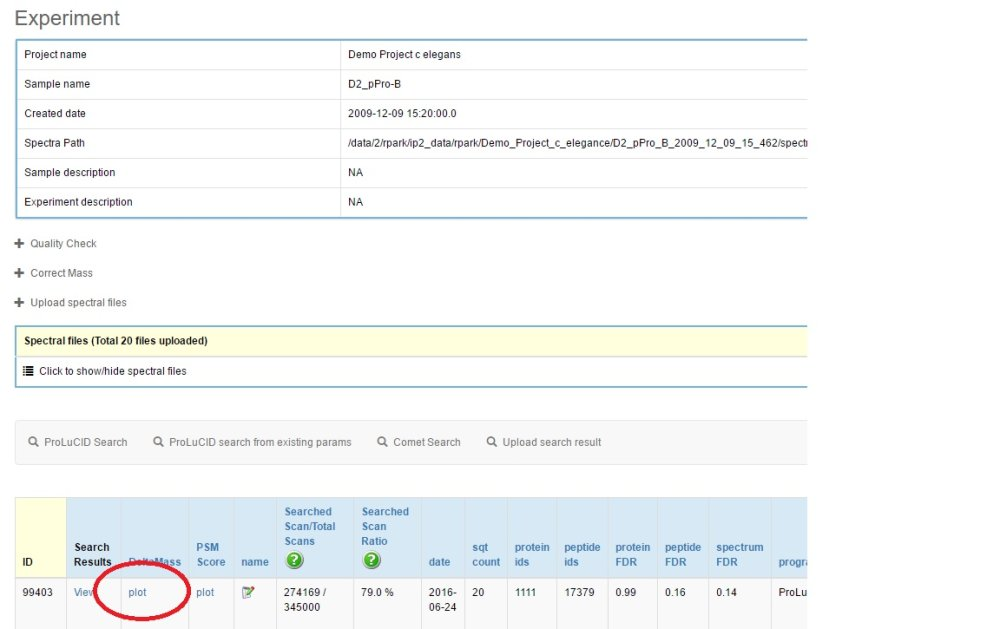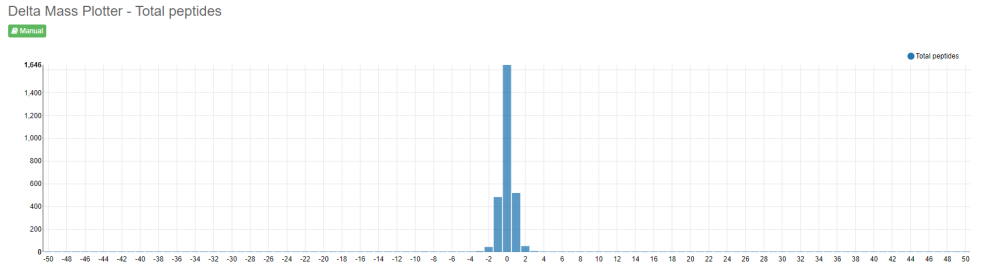PLOT DELTA MASS

Plot delta mass in ppm.

1. Click the “Plot” link in Delta Mass column on the search result page.#### Figure 7.4.1

2. The plot shows delta mass distribution. The x-axis is delta mass in ppm, and the y-axis is peptide count.

There are three different distribution graphs. The first one is the overall delta mass plot. The second and third ones are regular and PTM peptide delta mass distribution.#### Figure 7.4.2#### Figure 7.4.3